Categories

## Planetary Boundary Layer

The planetary boundary layer (PBL) is the layer of the atmosphere that interacts with the surface on a timescale of a day or less (Fig 21). It typically extends up to 1–3 km above the surface. The air above the PBL is called the free troposphere. The free troposphere has a general slow sinking motion, balancing the few locations where deep convection or frontal lifting injects PBL air to high altitudes. The compressional heating from this sinking air produces a semi-permanent subsidence inversion (Section 6) that caps the PBL and sharply restricts mixing between the PBL and the free troposphere.

Fig 21 Diurnal evolution of the planetary boundary layer (PBL) over land (a) and implications for chemical concentrations in surface air (b).

PBL dynamics plays an important role in determining the fate of chemicals emitted at the surface and the resulting concentrations in surface air. Vertical mixing driven by solar heating of the surface can drive large diurnal cycles of concentrations within the PBL. Venting of the PBL to the free troposphere is critical for global dispersal of chemicals.

Vertical mixing within the PBL is driven by turbulent eddies. These eddies are generated at the surface by the action of the wind on rough surface elements (mechanical turbulence) and by buoyancy (buoyant turbulence). Over land, sensible heating of the surface during the day generates buoyant plumes that may rise up to the base of the subsidence inversion. Conversely, nighttime cooling of the land surface produces stable conditions that dampen the mechanical turbulence. Over the oceans, the large heat capacity of the ocean minimizes this diurnal cycle of heating and cooling and the PBL remains neutral throughout the day.

Figure 21 shows the diurnal evolution of the PBL structure over land. At night, mechanical turbulence usually maintains a shallow, well-mixed layer typically 10–100 m deep called the surface layer. Above that altitude, the atmosphere is stable because of surface cooling; this is the residual layer. After sunrise, surface heating erodes the stable residual layer from below, producing an unstable mixed layer that grows over the morning hours to eventually reach the full depth of the PBL. Clouds may develop in the upper part; these are the familiar fair-weather cumuli and the corresponding layer is called the convective cloud layer (CCL). The CCL tends to have moderate stability due to the latent heat release from cloud condensation, resulting in some separation from the mixed layer. The depth of the mixed layer (excluding any CCL) is called the mixing depth. Suppression of surface heating at sunset causes rapid collapse of the mixed layer and the nighttime conditions return.

The diurnal variation of PBL structure has important implications for the diurnal evolution of chemical concentrations in surface air, as shown in Figure 21. An inert chemical continuously emitted at the surface will accumulate in surface air over the course of the night, leading to high concentrations. During morning the concentration will decrease as growth of the mixed layer causes dilution. By contrast, a chemical originating in the free troposphere and removed by deposition to the surface will be depleted in surface air over the course of the night, and replenished during morning by entrainment from aloft as the mixed layer grows.

Over the ocean there is no diurnal cycle of surface heating and cooling, and neutral conditions prevail where vertical mixing is driven by mechanical turbulence. The mixed layer is called the marine boundary layer (MBL) and typically extends to about 1 km altitude with no diurnal variation. It is often capped by a shallow cloud layer, either cumulus clouds or stratus, capped in turn by the subsidence inversion.

Entrainment of air from the free troposphere into the PBL and ventilation of PBL air to the free troposphere are important processes for atmospheric chemistry, connecting the surface to the global atmosphere. Ventilation generally takes place by weather events, such as frontal systems or deep convective updrafts that force boundary layer air to the free troposphere. Entrainment, by contrast, generally takes place as a slow, steady process involving the large-scale sinking of the atmosphere to compensate for the convective updrafts. Typical downward entrainment velocities at the top of the PBL are of the order of 0.1–1 cm s–1, and this replaces the PBL air on a timescale of days to a week.

Categories

## Barotropic and Baroclinic Atmospheres

barotropic atmosphere is one in which changes in air density are driven solely by changes in pressure. It is a good approximation in the tropics, where horizontal temperature gradients are small. In a barotropic atmosphere, isobaric (uniform pressure) surfaces coincide with isopycnic (uniform air density) surfaces. From the ideal gas law, they must also coincide with isothermal (uniform temperature) and isentropic (uniform potential temperature) surfaces. Since there is no temperature gradient on isobaric surfaces, the geostrophic wind is independent of height (see (38) and (39)). Under adiabatic conditions (dθ/dt = 0), air parcels remain on isentropic surfaces, and since no pressure gradient exists along these surfaces to drive atmospheric motions, no potential energy is available for conversion into kinetic energy.

Outside the tropics, where meridional temperature gradients are large (Figure 11), the temperature varies along the isobars, and the atmosphere is said to be baroclinic. Isobars and isentropes do not coincide. In this case, pressure gradients can drive adiabatic displacement along isentropic surfaces. Conversion of potential energy into kinetic energy becomes possible. Temperature gradients along isobars cause vertical shear in the geostrophic wind (see thermal wind equation), leading to a strong jet stream in the upper troposphere as discussed in Section 7. The axis of the jet stream is located in the 30°–60° latitudinal band characterized by a pronounced meridional temperature gradient separating cold and dense air of polar origin from warmer, less dense tropical air. In the presence of strong velocity shears, the jet stream may be unstable with respect to small perturbations, and disturbances may amplify, producing the so-called baroclinic instability.

Figure 13 illustrates baroclinic instability. The meridional gradient in temperature causes the isentropic surfaces (isentropes) to slope upward with increasing latitude. A poleward motion at constant altitude or with an upward slope shallower than the isentropes produces an unstable atmosphere even though the isentropes imply a vertically stable atmosphere (∂θ/z > 0). Despite the stable conditions, potential energy from the flow can be converted into kinetic energy. Baroclinic instabilities drive the development of mid-latitude cyclones and associated frontal systems.

Categories

## Geostrophic Balance

We now turn to the forces driving horizontal motions in the atmosphere. Horizontal pressure gradients resulting from differential heating produce motions directed from high- to low-pressure areas. A complication is that the Earth is a rotating sphere, where different points have different translational velocities in a fixed frame of reference. The useful frame of reference for us is one that rotates with the sphere, since we measure all air motions with respect to this frame of reference. From the perspective of this rotating frame of reference, any motion taking place in the fixed frame of reference (such as driven by a pressure-gradient force) will be deflected due to the rotation. The deflection accelerates the air parcel away from its original direction and thus behaves as a fictitious force, called the Coriolis force. The Coriolis force operates in three dimensions but is negligible in the vertical relative to the acceleration of gravity. It is of critical importance for large-scale motions in the horizontal direction.To understand the Coriolis effect, consider that the translational Earth’s rotation velocity VT (directed eastward)

VT = a Ω cos φ (29)

decreases with increasing latitude φ. With an Earth’s radius a = 6378 km and an angular rotation velocity Ω = 7.292 × 10–5 rad s–1 (or 2 π rad d–1), the velocity VT is 1672 km h–1 at the Equator and 836 km h–1 at 60° latitude. If we consider an air parcel that is displaced poleward in the northern hemisphere, starting from latitude φ1, the conservation of angular momentum in the absence of external forces requires that the product ρa V(φ) a cos φ remain constant at its initial value ρa VT1a cos φ1 during the displacement of the parcel. Here, V is the absolute eastward velocity of the air parcel in the fixed frame of reference, and ρa is the air density. Since VT(φ) decreases with latitude φ, this condition can only be fulfilled if, for an observer located at the Earth’s surface, the air parcel acquires a gradually increasing eastward velocity. For the same reason, an air parcel moving toward the Equator in the northern hemisphere will be displaced westward (see Figure 8).

Figure 8 Trajectory of an object (such as an air parcel) directed from the north pole toward the Equator at 90° W. (a) Case of a non-rotating planet. (b) Deflection of the trajectory toward the right due to the rotation of the Earth. The arrival point at the Equator is displaced to the west of the original target point.

The same Coriolis effect also applies to motions in the longitudinal direction. In that case it can be understood in terms of the centrifugal force exerted on air parcels in the rotating frame of reference of the Earth. An air parcel at rest at a given latitude is subject to a centrifugal acceleration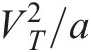that would make it drift toward the Equator were it not for the oblate geometry of the Earth (Figure 9). The resultant force of gravity (oriented toward the center of the Earth) and reaction (oriented normal to the surface) exactly cancels the centrifugal force, as shown in Figure 9. This should not be surprising considering that the oblate geometry is actually a consequence of the centrifugal force applied to the solid Earth. Consider now an eastward motion applied to the air parcel so that V > VT. This motion increases the centrifugal force and deflects the air parcel equatorward. Conversely, a westward motion with V < VT weakens the centrifugal force and deflects the air parcel poleward. In both cases the deflection is to the right in the northern hemisphere and to the left in the southern hemisphere.

Figure 9 Equilibrium of forces for an air parcel at rest in the frame of reference of the rotating Earth. The centrifugal force is directed away from the axis of rotation, gravity is directed toward the center of the Earth, and the reaction force is perpendicular to the surface. The centrifugal force would cause the air parcel to drift toward the Equator if the Earth were a perfect sphere. The oblate geometry of the Earth (greatly exaggerated for the purpose of this figure) results in equilibrium in the triangle of forces.Reproduced from Jacob. In summary, for an observer on the rotating Earth, air parcels moving horizontally are subject to a Coriolis force that is perpendicular to the direction of motion and proportional to the parcel’s velocity; this force deflects air parcels to the right in the northern hemisphere and to the left in the southern hemisphere. It can be shown that the corresponding Coriolis acceleration is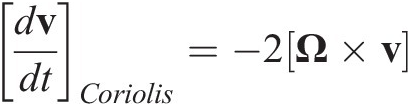(30)

where v represents the velocity vector in the rotating frame of reference and Ω is the Earth angular velocity vector directed from the south to the north pole. When expressed in Cartesian coordinates and considering the zonal and meridional wind components (u, v), the Coriolis acceleration becomes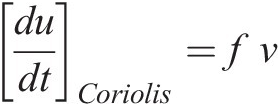(31)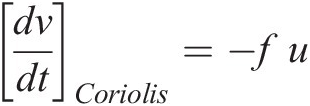(32)

where f = 2 Ω sin φ is the Coriolis parameter. It is positive in the northern hemisphere and negative in the southern hemisphere. Its amplitude increases with latitude. Thus, the Coriolis acceleration, which is zero at the Equator, increases with latitude and with the velocity of the flow. Its effect is substantial for large-scale motions (~1000 km, the synoptic scale).For an observer attached to the rotating Earth, the large-scale motions in the extratropical atmosphere can be represented by a balance between the Coriolis and the pressure-gradient forces, called the geostrophic approximation: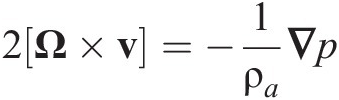(33)

or in a Cartesian projection (x and y being the geometric distances in the zonal and meridional directions, respectively)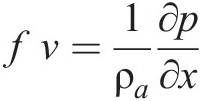(34)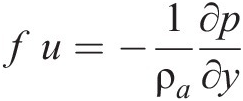(35)

From (33) we see that the geostrophic motions on a horizontal surface are parallel to the isobars (lines of constant pressure). In the northern hemisphere (f > 0), air parcels rotate clockwise around high pressure (anti-cyclonic) cells, and counter-clockwise around low pressure (cyclonic) cells (see Fig 10). The situation is reversed in the southern hemisphere (f < 0).

Fig 2.10 Flow of air in the northern hemisphere between anti-cyclonic (high) and cyclonic (low) regions. The motion originally directed from the high- to low-pressure cells is deflected to the right by the Coriolis force.When formulated using pressure rather than geometric altitude as the vertical coordinate, the geostrophic balance takes the form: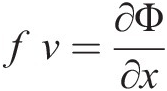(36)(37)

where Φ is the geopotential. Thus, on isobaric surfaces, the geostrophic motions follow the contours of the geopotential fields. Replacing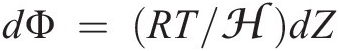yields the thermal wind equations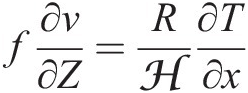(38)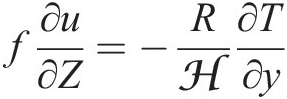(39)

These show that the vertical shear in the horizontal (constant pressure level) wind field is proportional to the horizontal temperature gradient. In both hemispheres, the zonal wind component u increases with height when temperature decreases with latitude and decreases with height when temperature increases with latitude (Fig 11). The strong decrease of temperature with latitude in the troposphere produces intense subtropical jet streams, seen in Fig 11 as westerly wind maxima centered at about 40° latitude and 10 km altitude.

Fig 11 Zonal mean temperature (a) and zonal wind velocity (b) as a function of latitude and altitude for January, from the COSPAR International Reference Atmosphere (CIRA).Reprinted with permission from Shepherd.

Near the surface, the geostrophic flow is modified by friction resulting from the loss of momentum as the flow encounters obstacles (vegetation, ocean waves, buildings, etc.). The friction force is directed in the direction opposite to the flow (slowdown of the wind), effectively weakening the Coriolis force. This deflects the flow toward areas of low pressure (or low geopotential areas on isobaric surfaces), as shown in Fig 12.

Figure 12 Winds around low- and high-pressure cells in the northern hemisphere. Geostrophic balance dominates aloft and the flow is directed along isobaric lines. Near the surface, friction deflects the flow toward low pressure.

Categories

## The Hydrostatic Approximation

The vertical variation of atmospheric pressure can be deduced from hydrostatic equilibrium,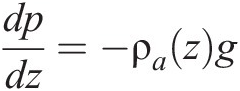(a)

which expresses that the downward gravitational force acting on a fluid parcel is balanced by an upward force exerted by the vertical pressure gradient that characterizes the fluid. Here g = 9.81 m s–2 is the acceleration of gravity, ρa [kg m–3] the mass density of air, p [Pa] the atmospheric pressure, and z [m] is the altitude above the surface, often referred to as the geometric altitude. Equation (a) assumes that the fluid parcel is in vertical equilibrium between the gravitational and pressure-gradient forces, or more broadly that any vertical acceleration of the air parcel due to buoyancy is small compared to the acceleration of gravity. This is called the hydrostatic approximation. It is a good approximation for global models, but not for small-scale models attempting to resolve strong convective motions.Because the atmosphere is thin relative to the Earth’s radius (6378 km), g can be treated as constant with altitude. From the ideal gas law, we can rewrite equation (a) as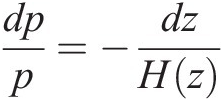(b)

where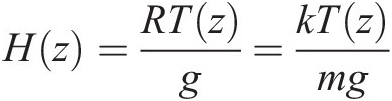(c)

is a characteristic length scale for the decrease in pressure with altitude z and is called the atmospheric scale height. Its value is 8 ± 1 km in the troposphere and stratosphere. Here

k = 1.38 × 10–23 J K–1

is Boltzmann’s constant,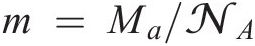is the mean molecular mass of air (28.97 × 10–3/6.022 × 1023 kg = 4.81 × 10–26 kg), and R = 287 J K–1 kg–1 is the specific gas constant for air. By integrating (b), one finds the vertical dependence of atmospheric pressure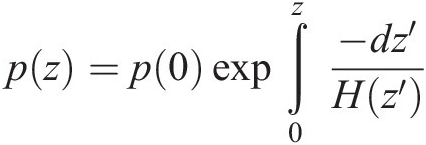(d)

where p(0) is the surface pressure. Approximating H as constant yields the simple expression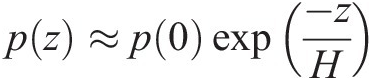(e)

which states that the air pressure decreases exponentially with altitude. Equation (e) is called the barometric law.The hydrodynamic equations of the atmosphere are often expressed by using the pressure p rather than the altitude z as the vertical coordinate. It is then convenient to define the log-pressure altitude Z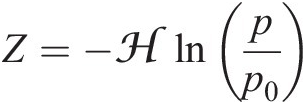(f)

as the vertical coordinate. Here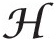is a constant “effective” scale height (specified to be 7 km) and p0 is a reference pressure (specified to be 1000 hPa). Thus Z depends solely on p. It is also convenient to introduce the geopotential Φ as the work required for raising a unit mass of air from sea level to geometric altitude z: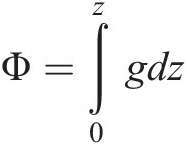(g)

where g includes dependences on altitude and latitude, the latter due to the non-sphericity of the Earth. g at Earth’s surface varies from 9.76 to 9.83 m s–2. The hydrostatic law relates Φ to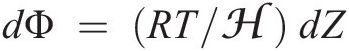.

The geopotential height is defined as Φ/go, where go = 9.81 m s–2 is a constant called the standard gravity. Movement along a surface of uniform geopotential height involves no change in potential energy, i.e., no conversion between potential and kinetic energy. Meteorological weather conditions aloft are often represented as contour maps of geopotential heights at a given pressure. As we will see in Section g, air motions tend to follow contour lines of geopotential heights, so this type of map is very useful.

Categories

## Atmospheric Humidity

Because of the high variability of water vapor in air, meteorologists like to use separate equations of state for dry air and water vapor. This is legitimate following Dalton’s law, which states that the total pressure of a mixture of gases is the sum of the partial pressures of its individual components. The equation of state for dry air is given by

pd = ρdRdT (6)

where the specific gas constant for dry air is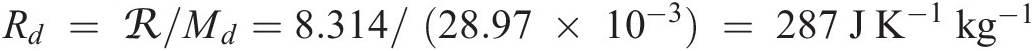. A similar equation can also be applied to water vapor (or any chemical constituent). The partial pressure of water vapor is commonly noted e and the equation of state is expressed by

e = ρwRwT

where ρw is the water vapor mass density [kg m–3] and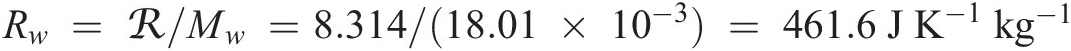is the specific gas constant for water vapor with a molar mass Mw of 18.01 × 10–3 kg mol–1.

Note that the total air pressure is

p = pd + e.

The volume mixing ratio Cw and mass mixing ratio μw of water vapor are expressed by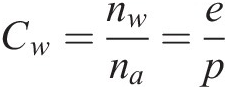and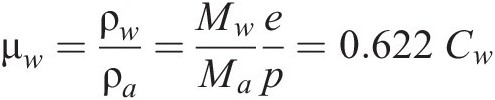where nw and na are the number densities [molecules m–3] of water and moist (total) air, respectively, Mw = 18.01 × 10–3 kg mol–1 is the molar mass of water, and Ma is the molar mass of moist air: Ma = (1 – Cw)Md + CwMw. Meteorologists conventionally call μw the specific humidity (and write it q). They instead define the water vapor mass mixing ratio rw as the ratio between the water vapor density ρw and the dry air density ρd, where

ρd = ρa  ρw: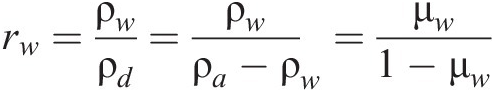The equation of state (6) for dry air can be applied to moist air if the temperature T is replaced by the virtual temperature Tv, the temperature at which dry air has the same pressure and density as moist air. Thus one writes

p = ρaRdTv

From the above equations, it follows that

or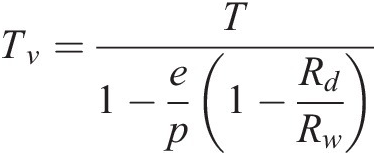with Rd/Rw = Mw/Md = 18/28.97 = 0.621. A good approximation to this expression is provided by

Tv ≈ (1 + 0.61 rw)T

Phase transitions of atmospheric water play a crucial role in meteorology. The relative humidity RH [percent] is expressed by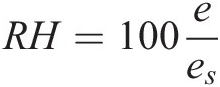where es is the saturation pressure at which water vapor is in equilibrium with the condensed phase (liquid or ice). For a saturated atmosphere (e = es), condensation and evaporation are in balance. One shows easily that the water mass mixing ratio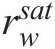corresponding to saturation is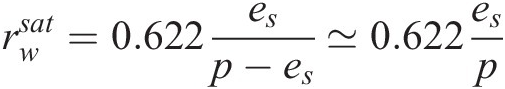Its value is inversely proportional to the total pressure and is a function of temperature because the saturation pressure es varies with temperature (see later). An atmosphere with e < es is called subsaturated while one with e > es is called supersaturated. A supersaturated atmosphere leads to cloud formation, contingent on the presence of suitable aerosol particles to provide pre-existing surfaces for condensation and overcome the energy barrier from surface tension. These particles are called cloud condensation nuclei (CCN) for liquid-water clouds and ice nuclei (IN) for ice clouds. Water-soluble particles greater than 0.1 μm in size are adequate CCN, and are sufficiently plentiful that liquid cloud formation takes place at supersaturations of a fraction of a percent. Ice nuclei are solid particles such as dust that provide templates for ice formation and are present at much lower concentrations than CCN. Because of the paucity of IN, clouds may remain liquid or mixed ice–liquid at temperatures as low as –40 °C; one then refers to the metastable liquid phase as supercooled.Phase equilibrium for water is defined by the phase diagram in Figure 5. Lines on this diagram represent equilibria between two phases. Equilibrium between vapor and condensed phases is expressed by the Clausius–Clapeyron equation

where L represents the latent heat of vaporization or sublimation [J kg–1]. Integration between a reference temperature T0 and temperature T yields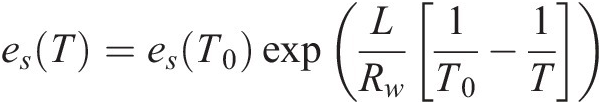where L can be approximated as constant. For vaporization of liquid water, L has a value of 2.50 × 106 J kg–1 at 0 °C and varies with temperature TC (in degrees Celsius) as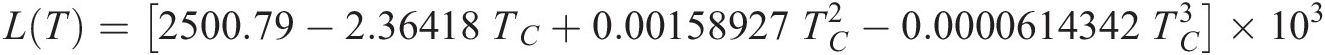For sublimation at 0 °C, L is 2.83 × 106 J kg–1. Latent heat is released to the atmosphere (warming) when clouds condense from the gas phase; conversely, latent heat is absorbed from the atmosphere (cooling) when clouds evaporate.

Figure 2.5 Phase diagram for water describing the stable phases present at equilibrium as a function of water vapor pressure and temperature. The thin line represents the metastable equilibrium between gas and liquid below 0 °C.Reproduced from Jacob

The thin line in the phase diagram of Figure 5 represents the metastable phase equilibrium between water vapor and liquid water at temperatures below 0 °C. This equilibrium is relevant to the atmosphere because of supercooling of liquid clouds. When ice crystals do form in such clouds, the water vapor at equilibrium with the ice is lower than that at equilibrium with the supercooled liquid; thus, the liquid cloud droplets evaporate, transferring their water to the ice crystals. This transfer of water can also take place by collision between the supercooled liquid cloud droplets and the ice crystals (riming). In either case, the resulting rapid growth of the ice crystals promotes precipitation. The heat release associated with the conversion from liquid to ice also adds to the buoyancy of air parcels, fostering further rise and additional condensation and precipitation. Such precipitation formation in mixed-phase clouds is known as the Bergeron process.

Categories

## Temperature, Pressure, and Density: The Equation of State

The state of the atmosphere is described by pressure p [Pa], temperature T [K], and chemical composition. Atmospheric pressure is sufficiently low that the ideal gas law is obeyed within 1% under all conditions. The equation of state can therefore be expressed as

pV = RT

where V [m3 mol–1] represents the molar volume of air, and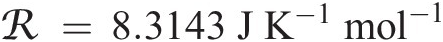is the universal gas constant. When expressed as a function of the number density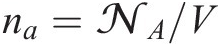[molecules m–3], where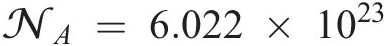molecules mol–1 is Avogadro’s number, this expression becomes

p = nakT

where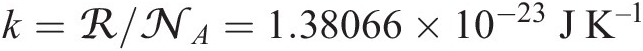is Boltzmann’s constant.The equation of state can also be expressed as a function of the mass density of air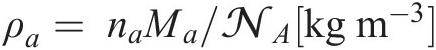p = ρaRT

where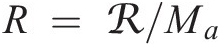is the specific gas constant for air and Ma [kg mol–1] is the molar mass of air.The molar mass of air is the weighted average of the mass of its components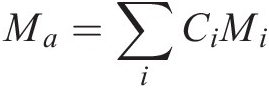where Ci and Mi are respectively the mole fraction (commonly called molar or volume mixing ratio) and the molar mass of constituent i. Since dry air can be closely approximated as a mixture of nitrogen N2 (with CN2 = 0.78), oxygen O2 (with CO2 = 0.21) and argon Ar (with CAr = 0.01), the molar mass for dry air is Md = 28.97 × 10–3 kg mol–1. Water vapor, which can account for up to a few percent of air in the lower troposphere, will make air slightly lighter.

Categories

## Vertical Structure of the Atmosphere

Figure 3 shows the mean vertical profile of atmospheric temperature. Atmospheric scientists partition the atmosphere vertically on the basis of this thermal structure. The lowest layer, called the troposphere, is characterized by a gradual decrease of temperature with height due to solar heating of the surface. It typically extends to 16–18 km in the tropics and to 8–12 km at higher latitudes. It accounts for 85% of total atmospheric mass. Heating of the surface allows buoyant motions, called convection, to transport heat and chemicals to high altitude. During this rise the water cools and condenses, leading to the formation of clouds. The process of condensation releases heat, providing additional buoyancy to the rising air parcels that can result in thunderstorms extending to the top of the troposphere. The mean decrease of temperature with altitude (called the lapse rate) in the troposphere is 6.5 K km–1, reflecting the combined influences of radiation, convection, and the latent heat release from water condensation.

Figure 3 Mean vertical profile of air temperature and definition of atmospheric layers.

The top of the troposphere, defined by a temperature minimum (190–230 K), is called the tropopause. The layer above, called the stratosphere, is characterized by increasing temperatures with height to reach a maximum of about 270 K at the stratopause located at 50 km altitude. This warming is due to the absorption of solar UV radiation by ozone. A situation in which the temperature increases with altitude is called an inversion. Because heavier air is overlain by lighter air, vertical motions are strongly suppressed. The stratosphere is therefore very stable against vertical motions. Exchange of air with the troposphere is restricted, and vertical transport within the stratosphere is very slow. The residence time of tropospheric air against transport to the stratosphere is 5–10 years, and the residence time of air in the stratosphere ranges from a year to a decade. In summer, the zonal (longitudinal) mean temperature distribution in the stratosphere is determined primarily by radiative processes (solar heating by ozone absorption and terrestrial cooling by CO2, water vapor, and ozone emission to space). In winter, radiation is weaker and the radiative equilibrium is perturbed by the propagation of planetary waves. This generates a large-scale meridional (latitudinal) circulation, called the Brewer–Dobson circulation, transporting air from low to higher latitudes.

The mesosphere extends from 50 km to the mesopause located at approximately 90–100 km altitude, where the mean temperature is about 160 K (120 K at the summer pole, which is the lowest temperature in the atmosphere). In this layer, where little ozone is available to absorb solar radiation, but where radiative cooling by CO2 is still effective, the temperature decreases again with height. Turbulence is frequent and often results from the dissipation of vertically propagating gravity waves, when the amplitude of these waves becomes so large that the atmosphere becomes thermally unstable.

The thermosphere above 100 km is characterized by a dramatic increase in temperature with height resulting primarily from the absorption of strong UV radiation by molecular oxygen O2, molecular nitrogen N2, and atomic oxygen O. Collisions become rare so that a stable population of ions can be sustained, producing a plasma (ionized gas). The temperature above 200 km reaches asymptotic values of typically 500 to 2000 K, depending on the level of solar activity (Figure 4). This asymptotic behavior reflects the small heat content and the high heat conductivity of this low air density region. The corresponding altitude is called the thermopause and varies from 250 to 500 km altitude. Atmospheric pressure is sufficiently low above 100 km that vertical transport of atmospheric species occurs primarily by molecular diffusion. This process tends to separate with height the different chemical species according to their respective mass. As a result, the relative abundance of light species like atomic oxygen, helium, and hydrogen increases with height relative to species like molecular nitrogen and oxygen. Molecular nitrogen dominates up to 180 km, while the prevailing constituent between 180 and about 700 km is atomic oxygen. Helium is the most abundant constituent between 700 km and 1700 km, and atomic hydrogen at higher altitudes. Above the thermopause, atoms follow ballistic trajectories because of the rarity of collisions. In this region of the atmosphere, light atoms (hydrogen) can overcome the forces of gravity and escape to space if their velocity is larger than a threshold value (escape velocity). At that point the atmosphere effectively merges with outer space.

Figure 4 Vertical distribution of the mean temperature for two levels of solar activity with emphasis on the upper atmosphere layers.

Air motions below 100 km are dominated by gravity and pressure forces, following the laws of hydrodynamics. Above 100 km, where ionization produces a plasma, the flow is affected by electromagnetic forces, and more complex equations from magneto-hydrodynamics must be applied. Aeronomy is the branch of science that describes the behavior of upper atmospheric phenomena (with emphasis on ionization and dissociation processes), while meteorology refers to the study of the lower levels of the atmosphere (with emphasis on dynamical and physical processes). The aeronomy literature has its own classification of atmospheric layers.

For example, it refers to the troposphere as the lower atmosphere, to the stratosphere and mesosphere as the middle atmosphere, and to the thermosphere as the upper atmosphere. It defines the homosphere below 100 km as the region where vertical mixing is sufficiently intense to maintain constant the relative abundance of inert gases, and the heterosphere above 100 km as the region where gravitational settling becomes sufficiently important for the relative concentration of heavy gases to decrease more rapidly than that of lighter ones. The atmospheric region above 1700 km is often called the geocorona. It produces an intense glow resulting from the fluorescence of hydrogen excited by the solar Lyman-α radiation at 122 nm. In another nomenclature, one distinguishes between the barosphere, where air molecules are bound to the Earth by gravitational forces, and the exosphere in which the air density is so small that collisions can be neglected. The lower boundary of the exosphere, called the exobase, is located at 400–1000 km.

Aeronomers refer to the ionosphere as the atmospheric region where ionization of molecules and atoms by extreme UV radiation (less than 100 nm) and energetic particle precipitation is a dominant process. Different ionospheric layers are distinguished: the D-region below 90 km altitude, the E-region between 90 and 170 km, the F-region between 170 and 1000 km, and the plasmasphere above 1000 km altitude. The region in which the magnetic field of the Earth controls the motions of charged particles is called the magnetosphere. Its shape is determined by the extent of the Earth’s internal magnetic field, the solar wind plasma, and the interplanetary magnetic field.

Categories

## Global Energy Budget

The main source of energy for the Earth system is solar radiation. The Sun emits radiation as a blackbody of effective temperature TS = 5800 K. The corresponding blackbody energy flux is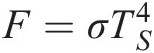(1)

where σ = 5.67 × 10–8 W m–2 K–4 is the Stefan-Boltzmann constant. This radiation extends over all wavelengths but peaks in the visible at 0.5 μm. The solar energy flux intercepted by the Earth’s disk (surface perpendicular to the incoming radiation) is 1365 W m–2. This quantity is called the solar constant and is denoted S. Thus, the mean solar radiation flux received by the terrestrial sphere is S/4 = 341 W m–2. A fraction α = 30% of this energy is reflected back to space by clouds and the Earth’s surface; this is called the planetary albedo. The remaining energy is absorbed by the Earth–atmosphere system. This energy input is compensated by blackbody emission of radiation by the Earth at an effective temperature TE. At steady state, the balance between solar heating and terrestrial cooling is given by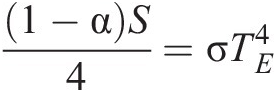(2)

The mean effective temperature deduced from this equation is TE = 255 K. It is the temperature of the Earth that would be deduced by an observer in space from measurement of the emitted terrestrial radiation. The corresponding wavelengths of terrestrial emission are in the infrared (IR), peaking at 10 μm. The effective temperature is 33 K lower than the observed mean surface temperature, because most of the terrestrial radiation emitted to space originates from the atmosphere aloft where clouds and greenhouse gases such as water vapor and CO2 absorb IR radiation emitted from below and re-emit it at a colder temperature. This is the essence of the greenhouse effect.

Figure 2 presents a more detailed description of the energy exchanges in the atmosphere. Of the energy emitted by the Earth’s surface (396 W m–2), only 40 W m–2 is directly radiated to space, while the difference (356 W m–2) is absorbed by atmospheric constituents. Thus, the global heat budget of the atmosphere must include the energy inputs resulting from (1) the absorption of infrared radiation by clouds and greenhouse gases (356 W m–2), (2) the latent heat released in the atmosphere by condensation of water (80 W m–2), (3) the sensible heat from vertical transport of air heated by the surface (17 W m–2), and (4) the absorption of solar radiation by clouds, aerosols, and atmospheric gases (78 W m–2). Of this total atmospheric heat input (532 W m–2), 199 W m–2 is radiated to space by greenhouse gases and clouds, while 333 W m–2 is radiated to the surface and absorbed. This greenhouse heating of the surface (333 W m–2) is larger than the heating from direct solar radiation (161 W m–2). At the top of the atmosphere, the incoming solar energy of 341 W m–2 is balanced by the reflected solar radiation of 102 W m–2 (corresponding to a planetary albedo of 0.30 with 23 W m–2 reflected by the surface and 79 W m–2 by clouds, aerosols and atmospheric gases) and by the IR terrestrial emission of 239 W m–2. Note that the system as described here for the 2000–2004 period is slightly out of balance because of anthropogenic greenhouse gases: A net energy per unit area of 0.9 W m–2 is absorbed by the surface, producing a gradual warming.

Categories

## Introduction

The atmosphere surrounding the Earth is a thin layer of gases retained by gravity (Fig). Table lists the most abundant atmospheric gases. Concentrations are expressed as mole fractions, commonly called mixing ratios. The principal constituents are molecular nitrogen (N2), molecular oxygen (O2), and argon (Ar). Their mixing ratios are controlled by interactions with geochemical reservoirs below the Earth’s surface on very long timescales. Water vapor is present at highly variable mixing ratios (10–6–10–2 mol mol–1), determined by evaporation from the Earth’s surface and precipitation. In addition to these major constituents, the atmosphere contains a very large number of trace gases with mixing ratios lower than 10–3 mol mol–1, including carbon dioxide (CO2), methane (CH4), ozone (O3), and many others. It also contains solid and liquid aerosol particles, typically 0.01–10 μm in size and present at concentrations of 101–104 particles cm–3. These trace gases and aerosol particles do not contribute significantly to atmospheric mass, but are of central interest for environmental issues and for atmospheric reactivity.fig: The Earth’s atmosphere seen from space, with the Sun just below the horizon. Air molecules scatter solar radiation far more efficiently in the blue than in the red. The red sunset color represents solar radiation transmitted through the lower atmosphere. The blue color represents solar radiation scattered by the upper atmosphere. Cloud structures are visible in the lowest layers.

## Table: Mixing ratios of gases in dry air

The mean atmospheric pressure at the Earth’s surface is 984 hPa, which combined with the Earth’s radius of 6378 km yields a total mass for the atmosphere of 5.14 × 1018 kg. As we will see, atmospheric pressure decreases quasi-exponentially with height: 50% of total atmospheric mass is found below 5.6 km altitude and 90% below 16 km. Atmospheric pressures are sufficiently low for the ideal gas law to be obeyed within 1% under all conditions. The global mean surface air temperature is 288 K, and the corresponding air density is 1.2 kg m–3 or 2.5 × 1019 molecules cm–3; air density also decreases quasi-exponentially with height.